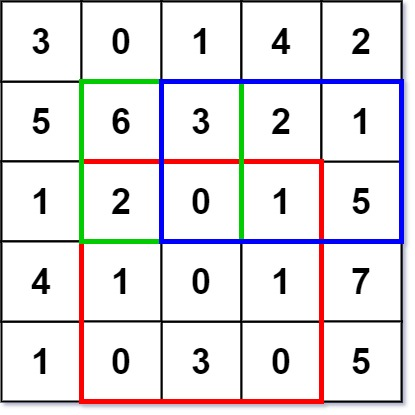# Leetcode 304 - Range sum query 2D - Immutable

Note:

• Use preSum.
• What is preSum in 2D? The sum of cells between [0, 0] and [i, j].
• How initialize preSum[][]?
•• Also we need to another an extra row and col and fill them with 0.
• How to find calc sum[i][j]?
• Just like how we initialized preSum but remember to add offset 1 to every row and col because there are an axtra row and col.

Question:

Given a 2D matrix matrix, handle multiple queries of the following type:

• Calculate the sum of the elements of matrix inside the rectangle defined by its upper left corner (row1, col1) and lower right corner (row2, col2).

Implement the NumMatrix class:

• NumMatrix(int[][] matrix) Initializes the object with the integer matrix matrix.
• int sumRegion(int row1, int col1, int row2, int col2) Returns the sum of the elements of matrix inside the rectangle defined by its upper left corner (row1, col1) and lower right corner (row2, col2).

Example:Code: